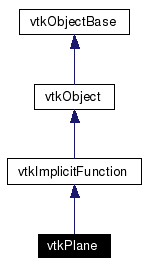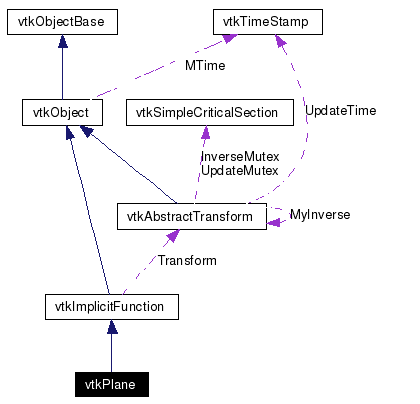# vtkPlane Class Reference

`#include <vtkPlane.h>`

Inheritance diagram for vtkPlane:[legend]
Collaboration diagram for vtkPlane:[legend]
List of all members.

## Detailed Description

perform various plane computations

vtkPlane provides methods for various plane computations. These include projecting points onto a plane, evaluating the plane equation, and returning plane normal. vtkPlane is a concrete implementation of the abstract class vtkImplicitFunction.

Examples:
vtkPlane (Examples)
Tests:
vtkPlane (Tests)

Definition at line 35 of file vtkPlane.h.
void Push (double distance)
static void ProjectPoint (double x, double origin, double normal, double xproj)

## Public Types

typedef vtkImplicitFunction Superclass

## Public Member Functions

virtual const char * GetClassName ()
virtual int IsA (const char *type)
void PrintSelf (ostream &os, vtkIndent indent)
double EvaluateFunction (double x)
double EvaluateFunction (double x, double y, double z)
void EvaluateGradient (double x, double g)
virtual void SetNormal (double, double, double)
virtual void SetNormal (double)
virtual double * GetNormal ()
virtual void GetNormal (double data)
virtual void SetOrigin (double, double, double)
virtual void SetOrigin (double)
virtual double * GetOrigin ()
virtual void GetOrigin (double data)

## Static Public Member Functions

static vtkPlaneNew ()
static int IsTypeOf (const char *type)
static vtkPlaneSafeDownCast (vtkObject *o)
static double Evaluate (double normal, double origin, double x)
static void GeneralizedProjectPoint (double x, double origin, double normal, double xproj)
static double DistanceToPlane (double x, double n, double p0)
static int IntersectWithLine (double p1, double p2, double n, double p0, double &t, double x)

vtkPlane ()
~vtkPlane ()

## Protected Attributes

double Normal 
double Origin 

## Member Typedef Documentation

 Reimplemented from vtkImplicitFunction. Definition at line 41 of file vtkPlane.h.

## Constructor & Destructor Documentation

 vtkPlane::vtkPlane ( ) ` [protected]`

 vtkPlane::~vtkPlane ( ) ` [inline, protected]`
 Definition at line 108 of file vtkPlane.h.

## Member Function Documentation

 static vtkPlane* vtkPlane::New ( ) ` [static]`
 Construct plane passing through origin and normal to z-axis. Reimplemented from vtkObject.

 virtual const char* vtkPlane::GetClassName ( ) ` [virtual]`
 Reimplemented from vtkImplicitFunction.

 static int vtkPlane::IsTypeOf ( const char * type ) ` [static]`
 Return 1 if this class type is the same type of (or a subclass of) the named class. Returns 0 otherwise. This method works in combination with vtkTypeRevisionMacro found in vtkSetGet.h. Reimplemented from vtkImplicitFunction.

 virtual int vtkPlane::IsA ( const char * type ) ` [virtual]`
 Return 1 if this class is the same type of (or a subclass of) the named class. Returns 0 otherwise. This method works in combination with vtkTypeRevisionMacro found in vtkSetGet.h. Reimplemented from vtkImplicitFunction.

 static vtkPlane* vtkPlane::SafeDownCast ( vtkObject * o ) ` [static]`
 Reimplemented from vtkImplicitFunction.

 void vtkPlane::PrintSelf ( ostream & os, vtkIndent indent ) ` [virtual]`
 Methods invoked by print to print information about the object including superclasses. Typically not called by the user (use Print() instead) but used in the hierarchical print process to combine the output of several classes. Reimplemented from vtkImplicitFunction.

 double vtkPlane::EvaluateFunction ( double x ) ` [virtual]`
 Evaluate plane equation for point x. Implements vtkImplicitFunction.

 double vtkPlane::EvaluateFunction ( double x, double y, double z ) ` [inline]`
 Evaluate function at position x-y-z and return value. You should generally not call this method directly, you should use FunctionValue() instead. This method must be implemented by any derived class. Reimplemented from vtkImplicitFunction. Definition at line 47 of file vtkPlane.h. References vtkImplicitFunction::EvaluateFunction().

 void vtkPlane::EvaluateGradient ( double x, double g ) ` [virtual]`
 Evaluate function gradient at point x. Implements vtkImplicitFunction.

 virtual void vtkPlane::SetNormal ( double , double , double ) ` [virtual]`
 Set/get plane normal. Plane is defined by point and normal.

 virtual void vtkPlane::SetNormal ( double  ) ` [virtual]`
 Evaluate function gradient at point x.

 virtual double* vtkPlane::GetNormal ( ) ` [virtual]`
 Evaluate function gradient at point x.

 virtual void vtkPlane::GetNormal ( double data ) ` [virtual]`
 Evaluate function gradient at point x.

 virtual void vtkPlane::SetOrigin ( double , double , double ) ` [virtual]`
 Set/get point through which plane passes. Plane is defined by point and normal.

 virtual void vtkPlane::SetOrigin ( double  ) ` [virtual]`
 Set/get point through which plane passes. Plane is defined by point and normal.

 virtual double* vtkPlane::GetOrigin ( ) ` [virtual]`
 Set/get point through which plane passes. Plane is defined by point and normal.

 virtual void vtkPlane::GetOrigin ( double data ) ` [virtual]`
 Set/get point through which plane passes. Plane is defined by point and normal.

 void vtkPlane::Push ( double distance )
 Translate the plane in the direction of the normal by the distance specified. Negative values move the plane in the opposite direction.

 static void vtkPlane::ProjectPoint ( double x, double origin, double normal, double xproj ) ` [static]`
 Project a point x onto plane defined by origin and normal. The projected point is returned in xproj. NOTE : normal assumed to have magnitude 1.

 static void vtkPlane::GeneralizedProjectPoint ( double x, double origin, double normal, double xproj ) ` [static]`
 Project a point x onto plane defined by origin and normal. The projected point is returned in xproj. NOTE : normal does NOT have to have magnitude 1.

 double vtkPlane::Evaluate ( double normal, double origin, double x ) ` [inline, static]`
 Quick evaluation of plane equation n(x-origin)=0. Definition at line 118 of file vtkPlane.h.

 double vtkPlane::DistanceToPlane ( double x, double n, double p0 ) ` [inline, static]`
 Return the distance of a point x to a plane defined by n(x-p0) = 0. The normal n must be magnitude=1. Definition at line 125 of file vtkPlane.h. References vtkPlaneAbs.

 static int vtkPlane::IntersectWithLine ( double p1, double p2, double n, double p0, double & t, double x ) ` [static]`
 Given a line defined by the two points p1,p2; and a plane defined by the normal n and point p0, compute an intersection. The parametric coordinate along the line is returned in t, and the coordinates of intersection are returned in x. A zero is returned if the plane and line do not intersect between (0<=t<=1). If the plane and line are parallel, zero is returned and t is set to VTK_LARGE_DOUBLE.

## Member Data Documentation

 double vtkPlane::Normal` [protected]`
 Definition at line 108 of file vtkPlane.h.

 double vtkPlane::Origin` [protected]`
 Definition at line 111 of file vtkPlane.h.

The documentation for this class was generated from the following file:

Generated on Tue Jan 22 00:07:27 2008 for VTK by1.4.3-20050530